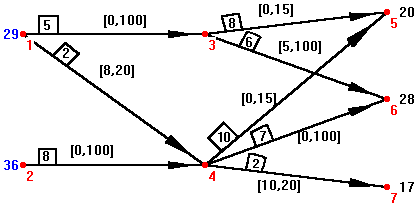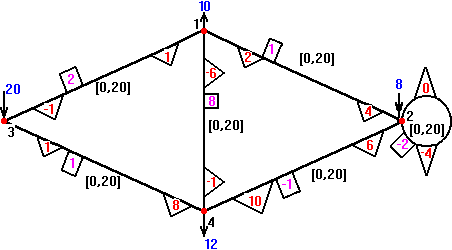## Prof. Dr. Ir. Arie M.C.A. Koster

subpages:
PhD thesis / DualNet /
DualNet
In 1995, I graduated on the software package DualNet. DualNet is a Windows application for solving the minimum cost flow problem. DualNet is made by order of the Departement of Statistics, Probability and Operations Research of the Faculty of Technical Mathematics and Informatics of Delft University of Technology.

The minimum cost flow problem (also mentioned as network-problem) is a well-known combinatorial optimization problem. The problem can be solved in polynomial time. An example of a min cost flow problem is given byThis problem is a pure network problem with arcs. You also have generalized network problems, with multipliers at the edges. An example of this problem is given byDualNet solves the problem by the dual simplex method for min cost flow problems. In this method each iterations is O(n), where n is the number of nodes in the network. In the dual simplex method the base of the problem is given by a tree (pure networks) or a collection of quasi-trees (generalized networks). A quasi-tree is a tree plus one more edge. In the program DualNet you can display these trees and the network on the screen in each iterations. If you choose to solve the problem direct, you only can display the network. In the network-window you can click on nodes and edges for more information about the costs, capacities, flow, etc. You also can move nodes in the window, to create your own interpretation of the network.

A DualNet-software-package (inclusive examples) is available here. DualNet isn't for commercial use. Since, the program starts in Dutch, you should read README.ENG before if you are not familiar with this language.